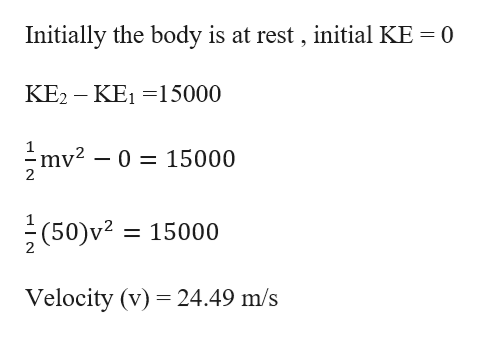# If a 50 kg object initially at rest is displaced 25 m by a constant net force of 600 N in the direction of motion of the object, then use the Work-Energy Theorem to find the final speed of the object.

Question
1 views

If a 50 kg object initially at rest is displaced 25 m by a constant net force of 600 N in the direction of motion of the object, then use the Work-Energy Theorem to find the final speed of the object.

check_circle

Step 1

Work done is equal to change in kinetic energy of the body .

Work done = (Force)*(displacem...help_outlineImage TranscriptioncloseInitially the body is at rest , initial KE 0 KE2 KE1 15000 mv2 - 0 = 15000 _ 2 (50)v2 15000 Velocity (v) 24.49 m/s fullscreen

### Want to see the full answer?

See Solution

#### Want to see this answer and more?

Solutions are written by subject experts who are available 24/7. Questions are typically answered within 1 hour.*

See Solution
*Response times may vary by subject and question.
Tagged in

### Work,Power and Energy